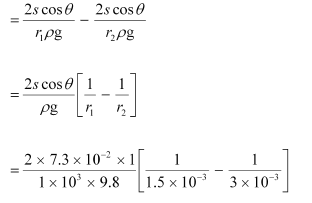## Pages

### Mechanical Properties of Fluids NCERT Solutions Class 11 Physics - Solved Exercise Question 10.30

Question 10.30:
Two narrow bores of diameters 3.0 mm and 6.0 mm are joined together to form a U-tube open at both ends. If the U-tube contains water, what is the difference in its levels in the two limbs of the tube? Surface tension of water at the temperature of the experiment is 7.3 × 10–2 N m–1. Take the angle of contact to be zero and density of water to be 1.0 × 103 kg m–3 (g = 9.8 m s–2).
Solution:
Diameter of the first bore, d1 = 3.0 mm = 3 × 10–3 m
Hence, the radius of the first bore, r1 = d1/ 2  =  1.5 X 10-3 m
Diameter of the first bore, d2 = 6.0 mm = 6 × 10–3 mm
Hence, the radius of the first bore, r2 = d2/ 2  =  3 X 10-3 m
Surface tension of water, s = 7.3 × 10–2 N m–1
Angle of contact between the bore surface and water, θ= 0
Density of water, ρ =1.0 × 103 kg/m–3
Acceleration due to gravity, g = 9.8 m/s2
Let h1 and h2 be the heights to which water rises in the first and second tubes respectively. These heights are given by the relations:
h1 = 2sCosθ / r1ρg      .....(i)
h2 = 2sCosθ / r2ρg      .....(ii)
The difference between the levels of water in the two limbs of the tube can be calculated as:= 4.966 X 10-3 m
= 4.97 mm
Hence, the difference between levels of water in the two bores is 4.97 mm.